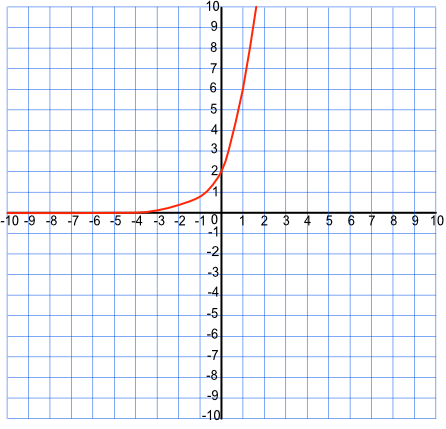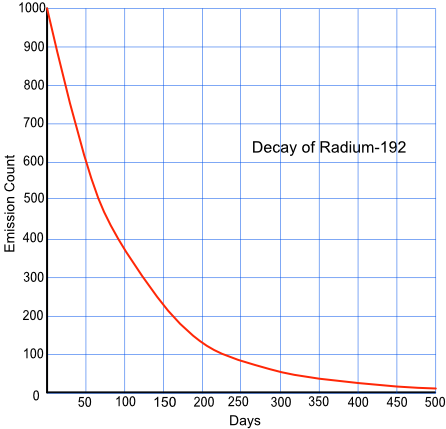Graphing Exponential Functions

# Graphing Exponential Functions

GCSE(H),

An exponential function is of the form nx, where n is a positive number, and which has been raised to a power. 2x, 5x and 7y are all exponentials. If the value of y increases as x increases, then this is known as exponential growth. If the value of y decreases as x increases, then this exponential decay.

## Examples

1.The sketch shows the equation y =abx, where a and b are constants. The graph passes through the points (0, 2) and (1, 6). What are the values of a and b?

Answer: a = 2 and b = 3

Working with the point (0, 2): x = 0, y = ab0. b0 = 1 therefore a = 2.

Working with the point (1, 6): 6 = 2b1 therefore b=3.

2. Radium-192 has a half-life of about 70 days. Using the table below, plot the graph of the number of emissions per second, and using the graph estimate the number of emissions after 100 days.

 Time (days) 0 70 140 210 280 350 Count /second 1000 500 250 125 63 31